Fingerings Home Octave 1: F  (C) Gb  (Db) G  (D) Ab  (Eb) A  (E) Bb  (F) B  (Gb) C  (G) Db  (Ab) D  (A) Eb  (Bb) E  (B) Octave 2+: F  (C) Gb  (Db) G  (D) Ab  (Eb) A  (E) Bb  (F) B  (Gb) C  (G) Db  (Ab) D  (A) Eb  (Bb) E  (B) F  (C) Gb  (Db) G  (D)Db (Ab) - 2nd Octave## Trills

Trill - From Eb   Trill - From D
Eb Db (alt)   D Db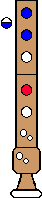Top

## Turned Trills

Turned Trill
Full step above, Full step below
Eb Db (alt) B Db (alt)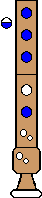Comments: The final alternate Db can easily break; you can use a regular Db instead, but it is more difficult.
Top

Turned Trill
Half step above; Full step below
D Db B (alt) Db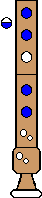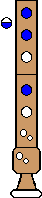Top

Turned Trill
Full step above; Half step below
Eb Db (alt) C DbTop

Turned Trill
Half step above; Half step below
D Db C (alt) Db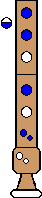Top

## Regular Turns

Turn
Full step above; Full step below
Eb (alt) Db B (alt) Db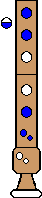Comments: You can start with a normal Eb, but the Db following it seems to speak more easily when you use the alternate Eb given.
Top

Turn
Half step above; Full step below
D Db B (alt) DbTop

Turn
Full step above; Half step below
Eb (alt) Db C (alt) DbComments: You can start with a normal Eb, but the Db following it seems to speak more easily when you use the alternate Eb given.
Top

Turn
Half step above; Half step below
D Db C (alt) Db